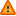FAQ/word - CBU statistics WikiYou should trust both wikis because the password could be read by the particular administrators.

Clear message
location: FAQ / word

## Producing equations in MS Word

MS Word supports some limited mathematical formulae components. These may be accessed by going to insert>Field and choosing Equations and Formulas as the category and Eq as the fieldname in the right hand window. Mathematical formulae syntax may then be placed after EQ in the descriptions box which is in the equations window you have just created.

Clicking the options button shows the available mathematical functions and their syntax available. These may be pasted into the descriptions box to form the equation. Functions available include subscripts and superscripts, root functions and fractions. They may be combined with ordinary text.

For example

`EQ \F(\R(,2)a,b\S( ,1)) `

produces the square root of 2 multiplied by a all divided by b with subscript of 1.

You can see this formula by typing the text in the above box into the descriptions box in the equation window in MS Word. You can't, though, just type the syntax into a MS Word file.

Note the space as the first argument of the subscript function, \S. In addition if Greek characters, used in some mathematical formulae, are required these may be found under insert>symbol.